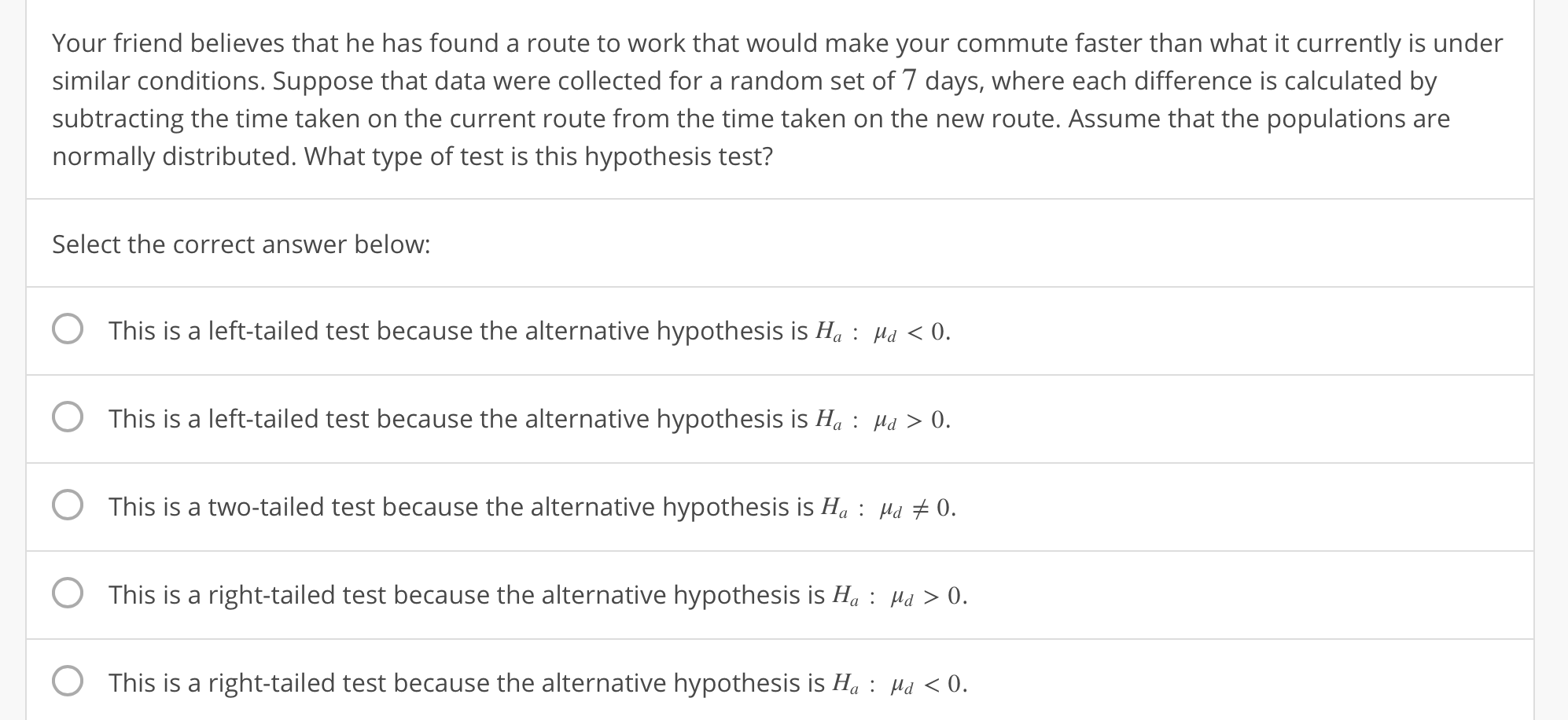# Your friend believes that he has found a route to work that would make your commute faster than what it currently is undersimilar conditions. Suppose that data were collected for a random set of 7 days, where each difference is calculated bysubtracting the time taken on the current route from the time taken on the new route. Assume that the populations arenormally distributed. What type of test is this hypothesis test?Select the correct answer below:O This is a left-tailed test because the alternative hypothesis is H: H 0O This is a left-tailed test because the alternative hypothesis is H0O This is a two-tailed test because the alternative hypothesis is H:Hu 0.0 This is a right-tailed test because the alternative hypothesis is Њ : d > 0.O This is a right-tailed test because the alternative hypothesis is H: H 0.

Question
132 viewshelp_outlineImage TranscriptioncloseYour friend believes that he has found a route to work that would make your commute faster than what it currently is under similar conditions. Suppose that data were collected for a random set of 7 days, where each difference is calculated by subtracting the time taken on the current route from the time taken on the new route. Assume that the populations are normally distributed. What type of test is this hypothesis test? Select the correct answer below: O This is a left-tailed test because the alternative hypothesis is H: H 0 O This is a left-tailed test because the alternative hypothesis is H0 O This is a two-tailed test because the alternative hypothesis is H:Hu 0. 0 This is a right-tailed test because the alternative hypothesis is Њ : d > 0. O This is a right-tailed test because the alternative hypothesis is H: H 0. fullscreen
check_circle

star
star
star
star
star
1 Rating
Step 1

Explanation:

The friend specifically believes that the new route is faster than the current route. This means that, according to the friend, the current route takes more time than the new route.

Now, the difference for each of the 7 days is obtained by subtracting the time taken on the current route, from that of the new route. So, if the friend’s claim is true and the current route really takes more time than the new one, this is subtracting a higher value from a lower value. Then the mean difference, µd, would be negative or less than 0.

Thus, the alternative hypothesis is:

Ha: µd < 0, that is, the new...

### Want to see the full answer?

See Solution

#### Want to see this answer and more?

Solutions are written by subject experts who are available 24/7. Questions are typically answered within 1 hour.*

See Solution
*Response times may vary by subject and question.
Tagged in

### Statistics